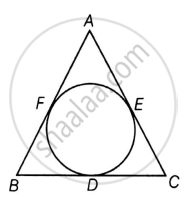# Let s denote the semi-perimeter of a triangle ABC in which BC = a, CA = b, AB = c. If a circle touches the sides BC, CA, AB at D, E, F, respectively, prove that BD = s – b. - Mathematics

Sum

Let s denote the semi-perimeter of a triangle ABC in which BC = a, CA = b, AB = c. If a circle touches the sides BC, CA, AB at D, E, F, respectively, prove that BD = s – b.

#### SolutionGiven: A triangle ABC with BC = a, CA = b and AB = c.

Also, a circle is inscribed which touches the sides BC, CA and AB at D, E and F respectively and s is semi- perimeter of the triangle

To Prove: BD = s - b

Proof: Given that

Semi Perimeter = s

Perimeter = 2s

Implies that

2s = AB + BC + AC  .....

As we know,

Tangents drawn from an external point to a circle are equal

So we have

AF = AE  ...... [Tangents from point A]

BF = BD  ...... [Tangents From point B]

CD = CE   ...... [Tangents From point C]

AF + BF + CD = AE + BD + CE

AB + CD = AC + BD

AB + CD + BD = AC + BD + BD

AB + BC - AC = 2BD

AB + BC + AC – AC – AC = 2BD

2s - 2AC = 2BD  ......[From 1]

2BD = 2s – 2b  .....[As AC = b]

BD = s – b

Hence Proved!

Concept: Concept of Circle - Centre, Radius, Diameter, Arc, Sector, Chord, Segment, Semicircle, Circumference, Interior and Exterior, Concentric Circles
Is there an error in this question or solution?

#### APPEARS IN

NCERT Mathematics Exemplar Class 10
Chapter 9 Circles
Exercise 9.4 | Q 2 | Page 110
Share